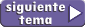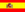home | más de estática | otros temas de Física | lecciones del maestro Ciruela | tonterías | @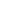NO ME SALEN
APUNTES Y EJERCICIOS RESUELTOS DE FÍSICA
Mecánica ClásicaStatic of the particle

Its called this way because it ignores or dismiss the rotation movements that a real body could have: it only cares about the displacement moves (which is the same criteria that we use in cinematics).

Statics is the study of equilibrium, and we understand that a body is in this situation as long as it has no acceleration. So we can state equilibrium as: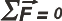In other words, it means that the resultant of all forces acting over the body, Res,  in other terms, the sum of all forces applied to it, ΣF, is equal to zero. So statics is just a particular case of dynamics (ΣF = m.a), in which acceleration is zero.

If we want to put this equilibrium condition down in equations, we have to remmember that this would be a vectorial relation. So if the forces acting on the body have the same position, we will only need one equation (ΣF = 0).

Although, if the forces applied on the body have different directions, but at the same time on a single plane, we will need two equations:

ΣFx = 0

ΣFy = 0

Of course, we should never forget to set the reference system, (X and Y axis), the same as we do in dynamics.

If forces act in diverse directions, not contained in a single plane, we will need 3 equations: one for each of the directions in our tridimensional refrence system (x, y, z).

Given that we're using particles as models, it's kind of obvious that all forces acting on a body, they concur. In the next chapter (rigid body) that is what is going to change.Lets see an example: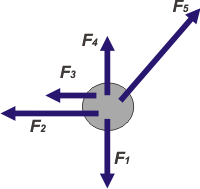Let's assume that there are 5 forces, which I've represented in the FBD on the left, applied on a body. Four of them point in perpendicular directions, and only one of them,F5, points at a whole different direction. Here is no doubt that the most confortable RS (for algebraic purposes) is the one that matches the direction of the first four forces (either way, i must reming you that any other RS is equaly valid, and will lead you to the same conclussions).

Once picked the RS, those forces (in this example, only F5) that won't match the directions of our RS, have to be replaced for them components in the directions of the RS.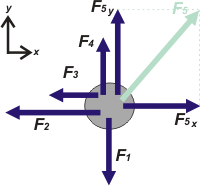See that in this second FBD you also have represented the RS. Don't forget to draw it! If they tell us that the body is in equilibrium, then we can state the next equations: dir. x:        F5x — F2 — F3 = 0 dir. y:         F5y + F4 — F1 = 0

 If during an exam you happen to forget to clearly state the Reference System, the teacher in charge will make sure that you pay for that.
Notice that in a proper representation, the forces won't be drawn one of top of the other (its understood that those are concurrent forces, meaning applied all at the same point).
Important Gossip:
• One of the coolest benefits of statics is that when an object (or a bunch of them) is in equilibrium, you can apply the statics relation to any of its parts, such as points where beams and columns meet, knots in a mesh, and any other place where forces concur.

Trick Questions:• A marble... Could be considered as a particle? And a hippo? What about a planet of the Solar System?

 Translated by Noelia Gattero. Some Rights Reserved. Not allowed to be copied without naming either the author or this source material. Last Updated oct-20. Buenos Aires, Argentina.